# Category Theory made easy with (ugly) pictures

27 Mar 2017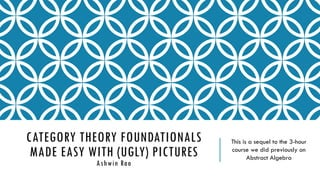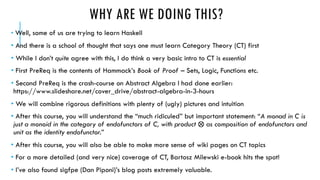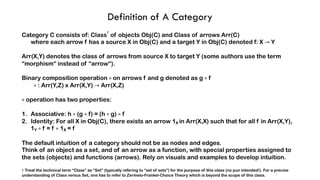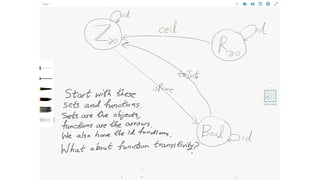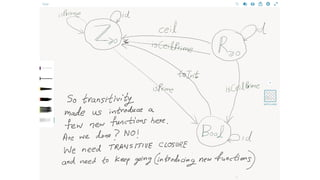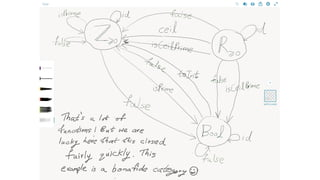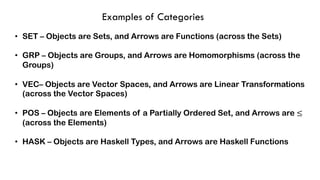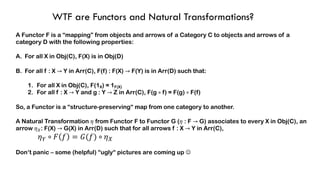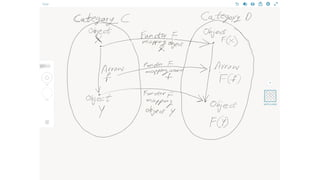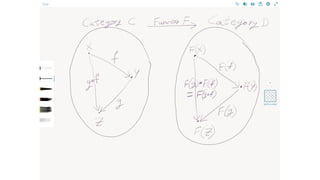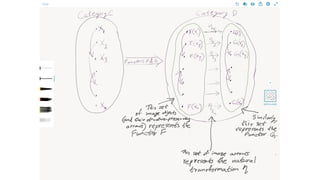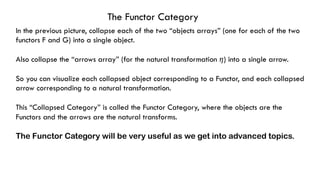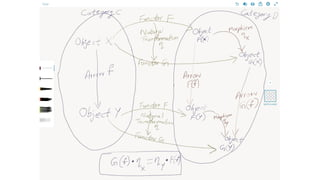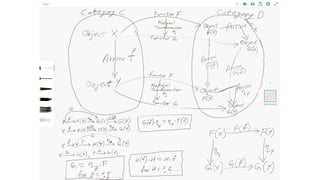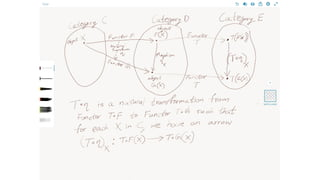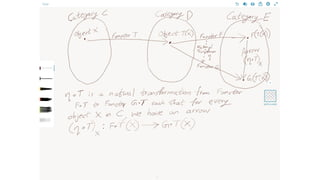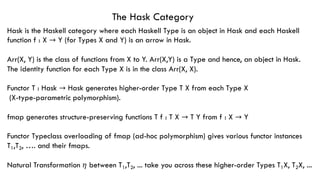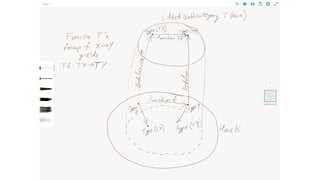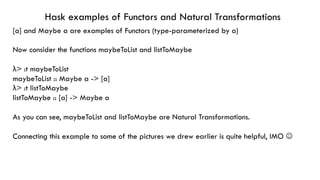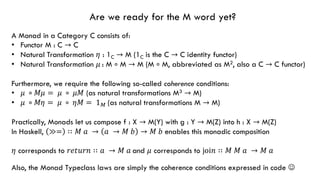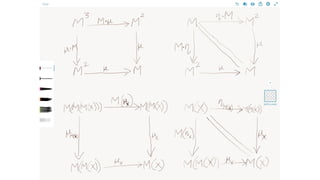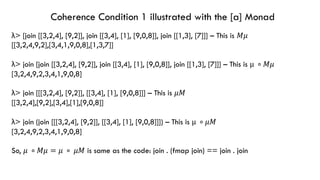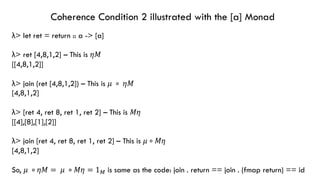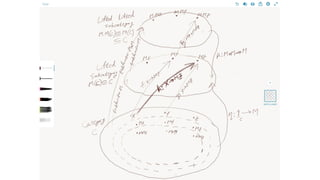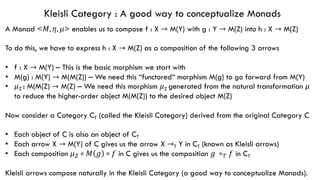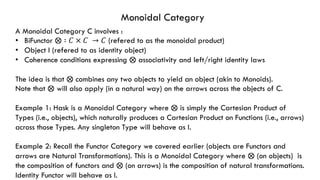1 sur 28

### Category Theory made easy with (ugly) pictures

• 1. CATEGORY THEORY FOUNDATIONALS MADE EASY WITH (UGLY) PICTURES Ashwin Rao This is a sequel to the 3-hour course we did previously on Abstract Algebra
• 2. WHY ARE WE DOING THIS? • Well, some of us are trying to learn Haskell • And there is a school of thought that says one must learn Category Theory (CT) first • While I don’t quite agree with this, I do think a very basic intro to CT is essential • First PreReq is the contents of Hammack’s Book of Proof – Sets, Logic, Functions etc. • Second PreReq is the crash-course on Abstract Algebra I had done earlier: https://www.slideshare.net/cover_drive/abstract-algebra-in-3-hours • We will combine rigorous definitions with plenty of (ugly) pictures and intuition • After this course, you will understand the “much ridiculed” but important statement: “A monad in C is just a monoid in the category of endofunctors of C, with product ⨂ as composition of endofunctors and unit as the identity endofunctor.” • After this course, you will also be able to make more sense of wiki pages on CT topics • For a more detailed (and very nice) coverage of CT, Bartosz Milewski e-book hits the spot! • I’ve also found sigfpe (Dan Piponi)’s blog posts extremely valuable.
• 3. Definition of A Category Category C consists of: Class † of objects Obj(C) and Class of arrows Arr(C) where each arrow f has a source X in Obj(C) and a target Y in Obj(C) denoted f: X → Y Arr(X,Y) denotes the class of arrows from source X to target Y (some authors use the term “morphism“ instead of “arrow“). Binary composition operation ∘ on arrows f and g denoted as g ∘ f ∘ : Arr(Y,Z) x Arr(X,Y) → Arr(X,Z) ∘ operation has two properties: 1. Associative: h ∘ (g ∘ f) = (h ∘ g) ∘ f 2. Identity: For all X in Obj(C), there exists an arrow 1X in Arr(X,X) such that for all f in Arr(X,Y), 1Y ∘ f = f ∘ 1X = f The default intuition of a category should not be as nodes and edges. Think of an object as a set, and of an arrow as a function, with special properties assigned to the sets (objects) and functions (arrows). Rely on visuals and examples to develop intuition. † Treat the technical term “Class” as “Set” (typically refering to “set of sets”) for the purpose of this class (no pun intended!). For a precise understanding of Class versus Set, one has to refer to Zermelo-Frankel-Choice Theory which is beyond the scope of this class.
• 7. Examples of Categories • SET – Objects are Sets, and Arrows are Functions (across the Sets) • GRP – Objects are Groups, and Arrows are Homomorphisms (across the Groups) • VEC– Objects are Vector Spaces, and Arrows are Linear Transformations (across the Vector Spaces) • POS – Objects are Elements of a Partially Ordered Set, and Arrows are ≤ (across the Elements) • HASK – Objects are Haskell Types, and Arrows are Haskell Functions
• 8. WTF are Functors and Natural Transformations? A Functor F is a “mapping” from objects and arrows of a Category C to objects and arrows of a category D with the following properties: A. For all X in Obj(C), F(X) is in Obj(D) B. For all f : X → Y in Arr(C), F(f) : F(X) → F(Y) is in Arr(D) such that: 1. For all X in Obj(C), F(1X) = 1F(X) 2. For all f : X → Y and g : Y → Z in Arr(C), F(g ∘ f) = F(g) ∘ F(f) So, a Functor is a “structure-preserving“ map from one category to another. A Natural Transformation 𝜂 from Functor F to Functor G (𝜂 : F → G) associates to every X in Obj(C), an arrow 𝜂(: F(X) → G(X) in Arr(D) such that for all arrows f : X → Y in Arr(C), 𝜂) ∘ 𝐹 𝑓 = 𝐺(𝑓) ∘ 𝜂( Don‘t panic – some (helpful) “ugly“ pictures are coming up J
• 12. The Functor Category In the previous picture, collapse each of the two “objects arrays” (one for each of the two functors F and G) into a single object. Also collapse the “arrows array” (for the natural transformation 𝜂) into a single arrow. So you can visualize each collapsed object corresponding to a Functor, and each collapsed arrow corresponding to a natural transformation. This “Collapsed Category” is called the Functor Category, where the objects are the Functors and the arrows are the natural transforms. The Functor Category will be very useful as we get into advanced topics.